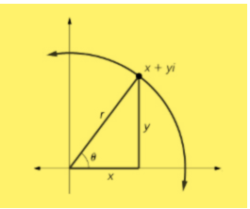# Value of i

The value of i is √-1. The imaginary unit number is used to express the complex numbers, where i is defined as imaginary or unit imaginary. We will explain here imaginary numbers rules and chart, which are used in Mathematical calculations. The basic arithmetic operations on complex numbers can be done by calculators. The imaginary number i is also expressed as j sometimes. Basically the value of imaginary i is generated, when there is a negative number inside the square root, such that the square of an imaginary number is equal to the root of -1. But when we take the cube of i, the value is -i. It is a solution to the quadratic equation or expression, x2+1 = 0, such as;

x2 = 0 – 1

x2 = -1

x = √-1

x = i

Therefore, an imaginary number is the part of complex number which we can write like a real number multiplied by the imaginary unit i, where i2 = -1. The imaginary number, when multiplied by itself, gives a negative value. For example, consider an imaginary number 3i, if multiplied by itself or if we take the square of 3i, gives 9i2 or we can write it as -9. Also, 0 is considered as both the real number and an imaginary number.

## What is the value of i?

We have learned till now the imaginary unit ‘i’ is used to represent the complex numbers which are non-real. But if we have to represent the complex numbers into a complete real form, we have to know the value of imaginary number ‘i’.

Basically, first, it was considered as there are no such facts as the imaginary number and real numbers. But when the complex number came into the picture, real numbers and imaginary numbers were differentiated. Some examples of complex numbers are;

1. 2+i6
2. 9-i7
3. i10

To solve such complex numbers, we have to know the value of i, which we can conclude from the expression, i2 = -1

If we take the square root on both sides, we get,

i2 = √-1

Or i = √-1

Therefore, the value if ‘i’ is √-1, which we cannot define, therefore, it is called an imaginary number.

### Value of i in Complex Number

The general form of the complex number can be expressed as;

X + i Y

Where X and Y are real numbers and i is an imaginary number.Squaring of the imaginary number i gives a negative value, but what if we square other numbers, like;

• Square of 1 = 12 = 1
• Square of -2 = (-2)2 = 4
• Square of 0.2 = (0.2)2 = 0.04

The above results do not give any negative number. Because as we know, the square of any real number is a positive number. But, the square of the imaginary unit is always negative and represents a non-real or complex number. Now we multiply the imaginary unit ‘i’ multiple times, let us see what result we get.

### Value of Powers of i

We know, i2 = -1, let us calculate the value of ‘i’ raised to the power other imaginary numbers.

 i3 i2 * i -i i4 i2 * i2 = -1 * -1 1 i5 i2 * i2 * i i i6 i2 * i2* i2 −1 i0 i1-1 = i1.i-1 = i1/i = i/i =1 1 i−1 1/-i = -i/(-i)2 = -i/1 −i i−2 1/i2 −1 i−3 1/i3=1/-i=i/(-i)2 i

From the above table you can see, the power of i repeats in a cycle, such that;

i4n = 1
i4n+1 = i
i4n+2= -1
i4n+3=-i

and so on.

Now let us solve some examples based on value of ‘i’.

### Solved Examples

Example 1: Solve the complex number √-4?

Solution: √-4 = √(-1 * 4) = 2√-1 = 2i

Example 2: Solve y2+1 = 0

Solution: y2+1 = 0

y= -1

y = √-1 = i

Check:

L.H.S = y2+1 = i2 + 1 = -1 + 1 = 0 (R.H.S)

Example 3: Find the value of √-3 + 1.

Solution: √-3 + 1

= √-1 √3 + 1

i√3 + 1

Example 4: Solve x2+16 = 0

Solution:  x2+16 = 0

x2= -16

x =√-16

x = i √16

x = i4

Check:

L.H.S = x2+16 = (i4)2 + 16 = i242 + 16 = -16 +16 = 0 (R.H.S)

Download BYJU’S – The learning App and experience a new method of learning with the help of visuals, graphics, pictures and understand the concepts easily.

Test your knowledge on Value Of I

1. But if the square root of 1 is 1 then the square root of -1 has to be 1 right?

• No square root of -1 is not 1 as their signs are different and square root of -1is not a real number but 1is a real no

• No
OK let
the root over of -4 = 2
then when we squaring both side
the ans will be -4 = 4
is it possible ?
definitely not
so root over of -1 = 1
then squaring both sides
ans => -1 = 1
that’s why it’s not possible.

2. so what is the value of i^5??

• i^5 = i^2.i^2.i = (-1)(-1)i = i

3. What does 2i equal then?

• i = √-1
2i = 2√-1 or √-4